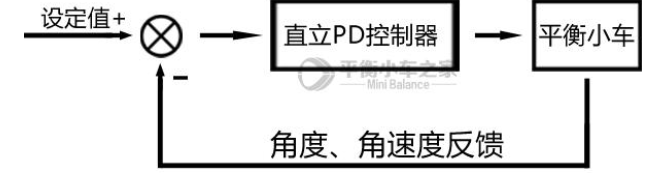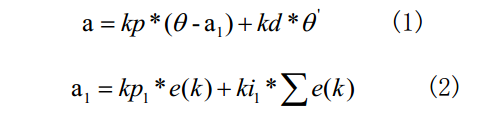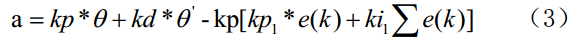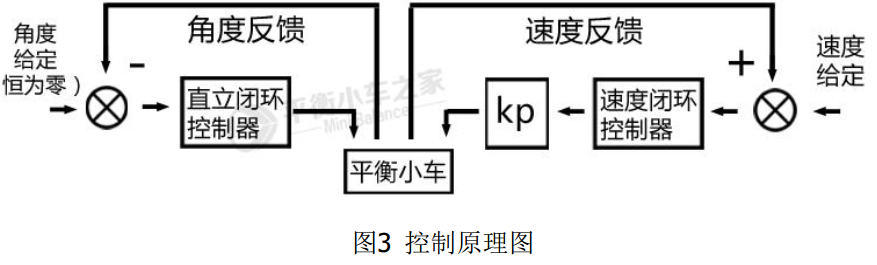# 平衡小车之PID算法

int balance(float Angle,float Gyro)//角度，角速度
{
float Bias,kp=300,kd=1;
int balance;
Bias=Angle-ZHONGZHI;       //求出平衡的速度中值
balance=kp*Bias+Gyro*kd;   //计算平衡控制的电机PWM PD控制，KP是p系数，KD是D系数
return balance;
}调试技巧：比例控制是引入了回复力，微分控制是引入了阻尼力，微分系数与转动惯量有关。

在小车质量一定的情况下，重心位置增高，因为需要的回复力减小，所以比例系数下降；转动惯量变大，所以微分控制系数增大。

在小车重心位置一定的情况下，质量增大，因为需要的回复力增大，比例控制系数增大；转动惯量变大，所以微分控制系数增大。

int velocity(int encoder_left,int encoder_right)
{
static float Velocity,Encoder_Least,Encoder,Movement;
static float Encoder_Integral;
float kp=80,ki=0.4;
//=============速度PI控制器=======================//
Encoder_Least =(Encoder_Left+Encoder_Right)-0;                    //获取最新速度偏差==测量速度（左右编码盘）
Encoder *= 0.8;                                                        //一阶低通滤波器
Encoder += Encoder_Least*0.2;                                        //一阶低通滤波器
Encoder_Integral +=Encoder;                                       //积分出位移  积分时间  10 ms
Encoder_Integral=Encoder_Integral-Movement;                       //接收遥控器数据，控制前进后退
if(Encoder_Integral>10000)      Encoder_Integral=10000;             //积分限幅
if(Encoder_Integral<-10000)    Encoder_Integral=-10000;              //积分限幅
Velocity=Encoder*kp+Encoder_Integral*ki;                          //速度控制
return Velocity;
}（1）为直立控制算法，（2）为速度控制算法，（3）为小车保持直立且速度为给定值的控制算法，由一个负反馈的直立PD控制器和一个正反馈的速度PI控制器组成。如下为控制原理图：